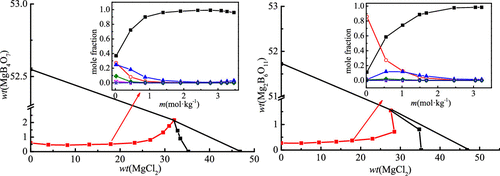Journal of Chemical & Engineering Data ( IF 2.298 ) Pub Date : 2020-01-07 , DOI: 10.1021/acs.jced.9b00852
Haiwen Ge; Hongjun Yang; Min WangThe solubility isotherms of the two ternary systems (MgCl2 + MgB4O7 + H2O) and (MgCl2 + Mg2B6O11 + H2O) were determined using the isothermal dissolution equilibrium method at 288.15 K. Solubilities, acidities, and densities of the liquid phase were determined in detail experimentally. According to the experimental data, the phase diagram and the relationship between density and composition were given. The results indicate that the ternary system (MgCl2 + MgB4O7 + H2O) is a simple saturated type, and double salt or solid solution are not formed at 288.15 K. There are one invariant point corresponding to (bischofite + magnesium borate), two isothermal dissolution curves, and two crystallization regions in the ternary system. There are one invariant point corresponding to bischofite and inderite, two isothermal dissolution curves, and two crystallization regions including bischofite and inderite in the ternary system (MgCl2 + Mg2B6O11 + H2O) at 288.15 K. It was found that the crystallization region area of magnesium borate was larger than that of bischofite, indicating that the solubility of bischofite was larger in the two systems. The distribution of polyborate species in magnesium borate isothermal dissolution curves varying with magnesium chloride molality was calculated by the Newton iteration method. The density data were calculated using the empirical formula, and the calculated results agree well with the experimental values.

down
wechat
bug